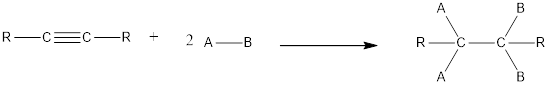Chapter 2, Problem 2.97EP### Organic And Biological Chemistry

7th Edition
STOKER + 1 other
ISBN: 9781305081079

#### Solutions

Chapter
Section### Organic And Biological Chemistry

7th Edition
STOKER + 1 other
ISBN: 9781305081079
Textbook Problem

# Supply the condensed structural formula of the product in each of the following alkyne addition reactions.(a)

Interpretation Introduction

Interpretation:

The condensed structural formula of the product that is formed in the given alkyne addition reaction has to be given.

Concept Introduction:

Chemical reaction in which an atom or a group of atoms are added to each carbon atom of a carbon‑carbon multiple bond in a hydrocarbon or hydrocarbon derivative is known as addition reaction.

In this reaction no atoms or group of atoms are removed. Instead the unsaturated bond is reduced to saturated bond. A general scheme for addition reaction of alkyne can be given as shown below,Explanation

The given reaction is,

CHCH + 2H2 Ni ?

By looking at the above reaction, the starting material is found to be an alkyne and hydrogen.  In presence of a metal catalyst such as Nickel, hydrogenation reaction takes place. The triple bond in alkyne gets reduced on addition of hydrogen atom. In the reaction two molecules of hydrogen is used. Hence, the triple bond will be converted into a single bond

(b)

Interpretation Introduction

Interpretation:

The condensed structural formula of the product that is formed in the given alkyne addition reaction has to be given.

Concept Introduction:

Chemical reaction in which an atom or a group of atoms are added to each carbon atom of a carbon‑carbon multiple bond in a hydrocarbon or hydrocarbon derivative is known as addition reaction.

In this reaction no atoms or group of atoms are removed. Instead the unsaturated bond is reduced to saturated bond. A general scheme for addition reaction of alkyne can be given as shown below,(c)

Interpretation Introduction

Interpretation:

The condensed structural formula of the product that is formed in the given alkyne addition reaction has to be given.

Concept Introduction:

Chemical reaction in which an atom or a group of atoms are added to each carbon atom of a carbon‑carbon multiple bond in a hydrocarbon or hydrocarbon derivative is known as addition reaction.

In this reaction no atoms or group of atoms are removed. Instead the unsaturated bond is reduced to saturated bond. A general scheme for addition reaction of alkyne can be given as shown below,(d)

Interpretation Introduction

Interpretation:

The condensed structural formula of the product that is formed in the given alkyne addition reaction has to be given.

Concept Introduction:

Chemical reaction in which an atom or a group of atoms are added to each carbon atom of a carbon‑carbon multiple bond in a hydrocarbon or hydrocarbon derivative is known as addition reaction.

In this reaction no atoms or group of atoms are removed. Instead the unsaturated bond is reduced to saturated bond. A general scheme for addition reaction of alkyne can be given as shown below,### Still sussing out bartleby?

Check out a sample textbook solution.

See a sample solution

#### The Solution to Your Study Problems

Bartleby provides explanations to thousands of textbook problems written by our experts, many with advanced degrees!

Get Started

#### Find more solutions based on key concepts